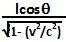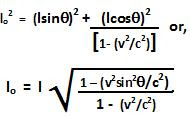## Sunday, September 20, 2009

### Irodov Problem 1.342Let lo be the original length of the rod. The rod moves at a velocity v and at an anglewith respect to the stationary frame and has a length l. If the rod slowed down to a velocity zero, it would have a length lo but it would not be aligned at an angle. The rod would have shrunk in the horizontal direction (direction of motion) but not in the vertical direction (perpendicular to the direction of motion). So the vertical component of the length of the rod remains unchanged whether the rod is moving or not at. The horizontal component of the rod however will ne longer when the rod is at rest and equal to. The rest length will thus be,